## NCERT Solutions for Class 12 Maths Chapter 13 Probability Ex 13.4

The topics and sub-topics included in Chapter 13 Probability the following:

 Section Name Topic Name 13 Probability 13.1 Introduction 13.2 Conditional Probability 13.3 Multiplication Theorem on Probability 13.4 Independent Events 13.5 Bayes’ Theorem 13.6 Random Variables and its Probability Distributions 13.7 Bernoulli Trials and Binomial Distribution

NCERT Solutions for Class 12 Maths Chapter 13 Probability 13.4 are part of NCERT Solutions for Class 12 Maths. Here we have given Class 12 Maths NCERT Solutions Probability Ex 13.4

Class 12 Maths Probability Solutions

Question 1.
State which of the following are not the probability distributions of a random variable. Give reasons for your answer.Solution:Question 2.
An urn contains 5 red and 2 black balls. Two balls are randomly drawn. Let X represent the number of black balls. What are the possible values of X ?. Is X a random variable?
Solution:Question 3.
Let X represent the difference between the number of heads and the number of tails obtained when a coin is tossed 6 times. What are possible values of X?
Solution:Question 4.
Find the probability distribution of
(a) number of heads in two tosses of a coin.
(b) number of tails in the simultaneous tosses of three coins.
(c) number of heads in four tosses of a coin.
Solution: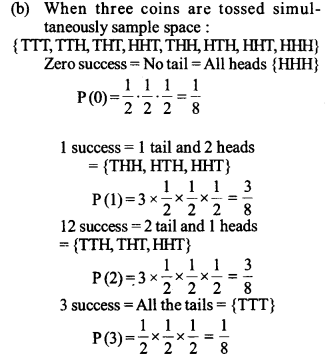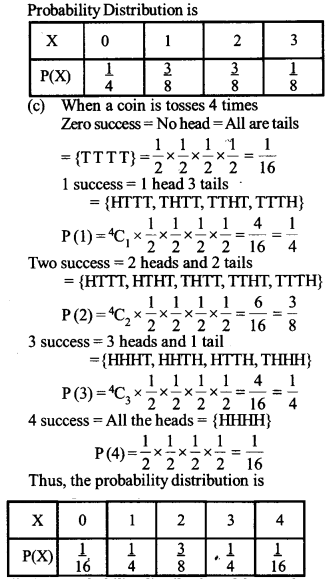Question 5.
Find the probability distribution of the number of successes in two tosses of a die where a success Is defined as
(i) number greater than 4
(ii) six appears on at least one die
Solution: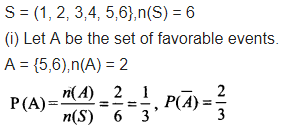Question 6.
From a lot of 30 bulbs which include 6 defectives, a sample of 4 bulbs is drawn at random with replacement Find the probability distribution of the number of defective bulbs.
Solution: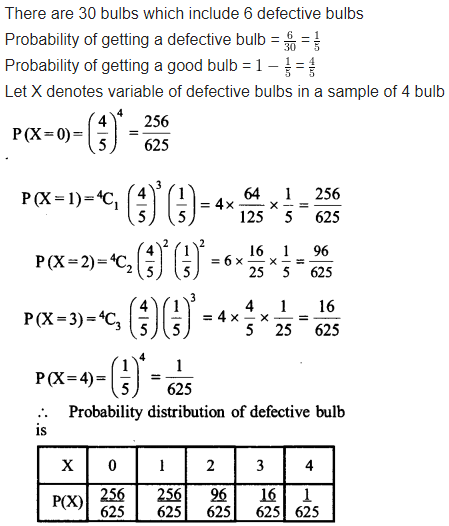Question 7.
A coin is biased so that the head is 3 times as likely to occur as tail. If the coin is tossed twice, find the probability distribution of number of tads.
Solution: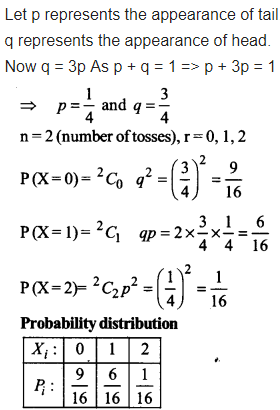Question 8
A random variable X has the following probability distribution:Determine
(i) k
(ii) P(X<3) (iii) P(X>6)
(iv) P(0<X<3)
Solution:Question 9.
The random variable X has a probability distribution P (X) of the following form, where k is some number(a) Determine the value of k
(b) FindP(X<2),P (X≤2), P(X≥2)
Solution: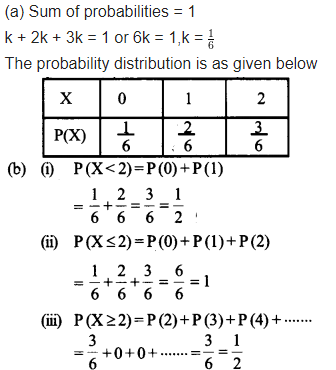Question 10.
Find the mean number of heads in three tosses of a fair coin.
Solution: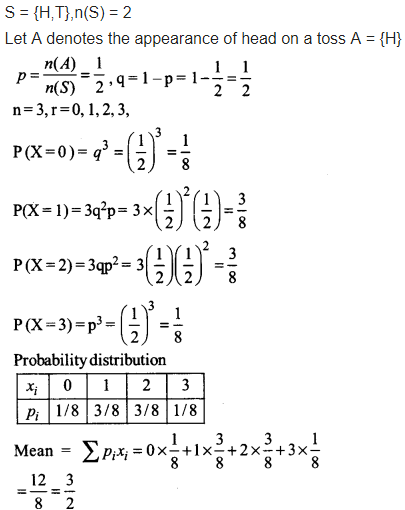Question 11.
Two dice are thrown simultaneously. If X denotes the number of sixes, find the expectation of X.
Solution: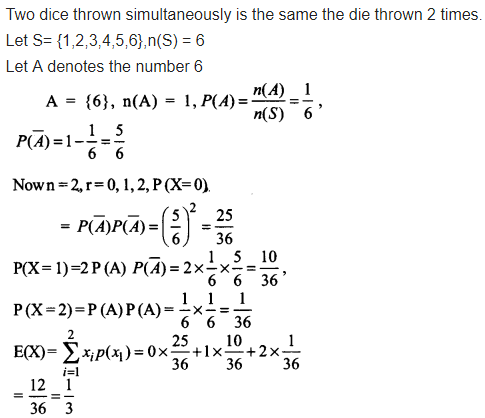Question 12.
Two numbers are selected at random (without replacement) from the first six positive integers. Let X denote the larger of the two numbers obtained. Find E (X)
Solution: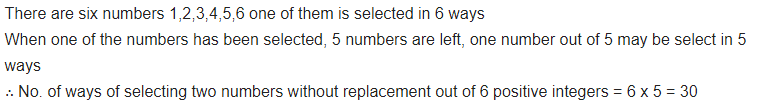Question 13.
Let X denote the sum of the numbers obtained when two fair dice are rolled. Find the variance and standard deviation of X.
Solution: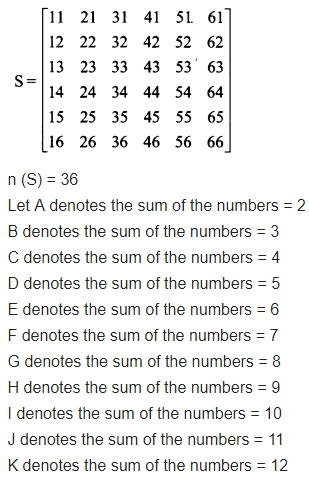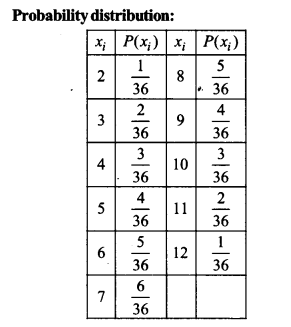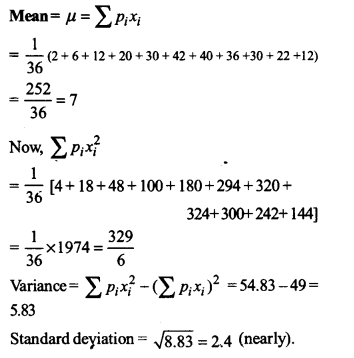Question 14.
A class has 15 students whose ages are 14,17, 15,14,21,17,19,20,16,18,20,17,16,19 and 20 years. One student is selected in such a manner that each has the same chance of being chosen and the age X of the selected student is recorded.What is the probability distribution of the random variable X ? Find mean, variance and standard deviation of X?
Solution: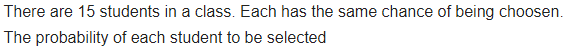Question 15.
In a meeting, 70% of the members favour and 30% oppose a certain proposal. A member is selected at random and we take X = 0, if he opposed, and X = 1, if he is in favour Find E (X) and Var (X).
Solution: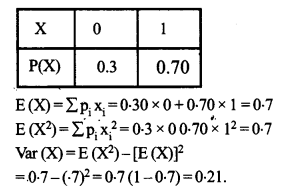Choose the correct answer in each of the following:

Question 16.
The mean of the number obtained on throwing a die having written 1 on three faces, 2 on two faces and 5 on one face is
(a) 1
(b) 2
(c) 5
(d) $$\frac { 8 }{ 3 }$$
Solution:Question 17.
Suppose that two cards are drawn at random from a deck of cards. Let X be the number of aces obtained. What is the value of E (X)?
(a) $$\frac { 37 }{ 221 }$$
(b) $$\frac { 5 }{ 13 }$$
(c) $$\frac { 1 }{ 13 }$$
(d) $$\frac { 2 }{ 13 }$$
Solution:We hope the given NCERT Solutions for Class 12 Maths Chapter 13 Probability Exercise 13.4 will help you. If you have any query regarding Class 12 Maths NCERT Solutions Probability Ex 13.4, drop a comment below and we will get back to you at the earliest.